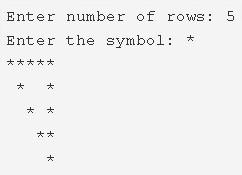# C Program Hollow Inverted Mirrored Right Triangle

C Program to print a hollow inverted mirrored right triangle star pattern – In this article, we will brief in on the several methods used to print a hollow inverted mirrored right triangle star pattern in C programming.

Suitable examples and sample programs have also been added so that you can understand the whole thing very clearly. The compiler has also been added with which you can execute it yourself.

The various ways used in this piece are as follows:

• Using For Loop
• Using While Loop
• Using Do-While Loop

As you all know, a right triangle is a triangle whose one of the angles is equal to 90 degrees. The other angles make up a sum of 90 degrees as well.

The sum of the square of the sides adjacent to the right angle is equal to the square of the side that is opposite to the right angle.

This is how a hollow inverted mirrored right triangle looks like:As you can see, you need to input the number of rows beforehand. Accordingly, it will print out the right triangle for the same number of rows.

You need to assign the symbol as well with which your triangle will be printed with.

Thus, the multiple methods used to print a hollow inverted mirrored right triangle star pattern are as follows:

## Using For Loop

1. Using scanf() function read the ‘entered number of rows’, read the character using getchar() function and store those values into the variables n, ch.

2) The outer for loop iterates through rows with the structure for(i=n;i>0;i–).

a) The 1st inner for loop for(j=n-i;j>o;j–) iterates through columns.

It prints space.

b) For i=1 or n(1st row or nth row)

The 2nd inner for loop iterates through columns with the structure for(j=1;j<=i;j++).

It prints the symbol which is entered by the user.

c) For i!=1 or i!=n(other than 1st row,nth row).

The 3rd inner for loop iterates through columns with the structure for(j=1;j<=i;j++)

It prints symbol if j=1 or i. Otherwise, it prints space.

3)Cursor comes to next line for each iteration of outer for loop.

Output:

### Using While Loop

1. i=n, The outer while loop iterates through rows until the condition i>0 becomes false.

2) j=n-i, The 1st inner while loop iterates through columns until the condition j>0 fails.

Prints space,j value decreased by 1. The 1st inner loop again checks the condition.

3) For 1st row, nth row

The 2nd inner while loop iterates through columns until the condition j<=i becomes false.

Prints symbol.j value increased by 1. The 2nd inner loop again checks the condition.

4) For other than the 1st row, last row

The 3rd inner while loop iterates through columns until the condition j<=i becomes false.

Prints symbol if j=1 or i. Otherwise, it prints space.

j value increased by 1. The 3rd loop again checks the condition.

5) Cursor comes to next line, i value decreased by 1. The outer while loop again checks the condition.

Output:

#### Using Do-While Loop

1. i=n, The outer do-while loop iterates through rows.

j=n-i, The while loop iterates until the condition j>0 fails.

Prints space,j value decreased by 1.

2) For 1sr row, nth row

j=1, The 1st inner do-while loop iterates through columns.

Prints symbol,j value increased by 1.

Checks the condition j<=i, if the condition is true then loop iterates again. Repeat until the condition becomes false.

3) For other than the 1st row, last row

The 2nd inner do-while loop iterates through columns.

It prints symbol if j=1 or i. Otherwise, it prints space.

j value increased by 1.

Checks the condition j<=i, if the condition is true then loop iterates again. Repeat until the condition becomes false.

4) Cursor comes to next line.i value decreased by 1.

5) Checks the condition i>0, if the condition is true then the loop iterates again.Repeats until the condition becomes false.

Output:
x

## C Program To Print Number Of Days In A Month | Java Tutoring

C program to input the month number and print the number of days in that ...